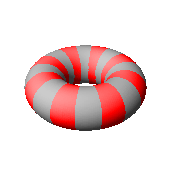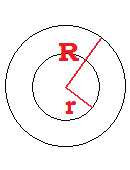# Surface Area and Volume of a Torus

Torus Calculator
Outer Radius R =
Inner Radius r ="Torus" is the technical mathematical name for the three-dimensional shape of a doughnut. A torus is generated by rotating a ring about an axis outside of the ring. To find the surface area and volume of a doughnut, you need to know the outer radius R and the inner radius r, i.e., the radius of the doughnut hole. You can apply the formulas described below, or you can use the convenient torus calculator.

### Volume of a Torus

If the outer radius of the doughnut is R and the inner radius is r, then the volume of the solid is given by the equation

Volume = (1/4)(pi2)(R2 - r2)(R - r).

Recall that pi is the constant 3.14159265358...

### Surface Area of a Torus

The surface area formula for a torus is

Surface Area = (pi)2(R2 - r2).

Example

Suppose a bagel is 5 inches wide and the hole is 2 inches across. Then R = 2.5 and r = 1. The volume is thus

(1/4)(pi2)(2.52 - 12)(2.5 - 1)
= 19.43 cubic inches.

The surface area is

(pi)2(2.52 - 12)
= 51.82 square inches.